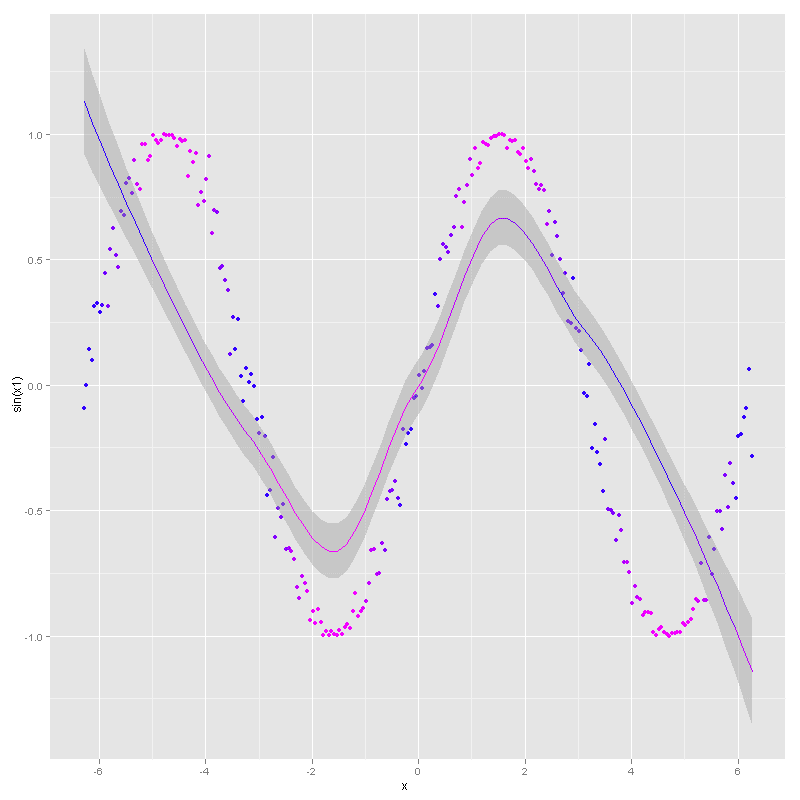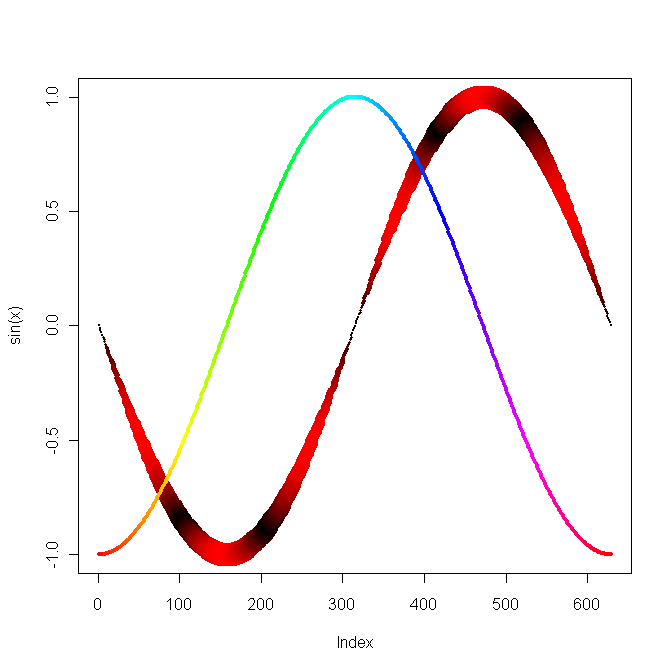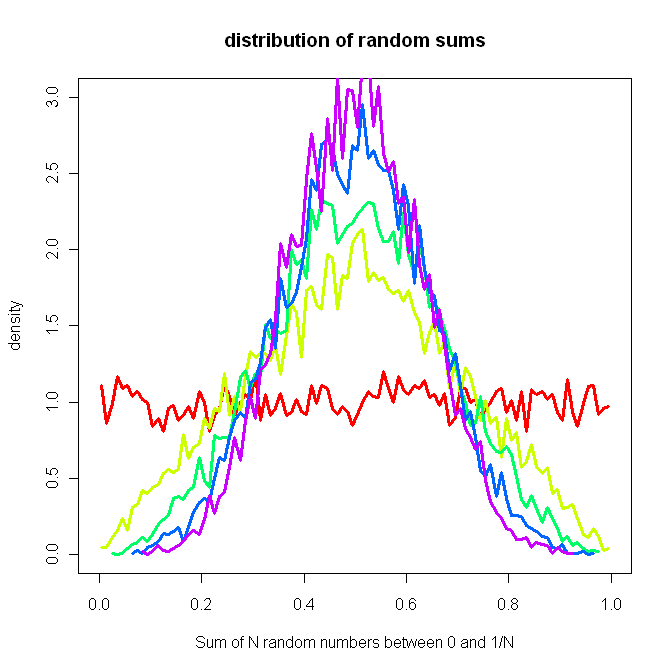## Thursday, 19 November 2009

### R and Datetimes and stuff

You may have figured out at this point that these posts' primary purpose is so I don't have to figure out this stuff again if I stop using R for 6 months.

Anyway, if you've got some strings and you want dates, use`as.Date(x)`

But if you want datetimes (i.e. don't want to lose the time component), use `as.POSIXct(x)`

Also, subset is nice:`subset(dat1, (t > '2009-01-01') & (t < '2009-01-05'))`

Create a frame: `#make up some datax = seq(-2*pi, 2*pi, by = 0.05)#create the framedatx = data.frame(x=x)#add another columndatx\$sinx = sin(datx\$x)#show the columnsnames(datx)#gives:  "x" "sinx"`
See also http://www.statmethods.net/input/datatypes.html for a nice brief intro to R's different structures.

### ggplot2 is awesome

I like R, but R+ggplot2 is awesome.`library("ggplot2")x = seq(-2*pi, 2*pi, by = 0.05)x1 = x + rnorm(length(x))/10qplot(x, sin(x1), color=rgb(abs(sin(x)),0,1), geom = c("point", "smooth"))`

Requires that you `install.packages()` ggplot2, of course.

I'm currently working through Getting started with qplot (pdf).

## Wednesday, 18 November 2009

### The R Project for Statistical Computing

I've been playing with The R Project for Statistical Computing. I quite like it.

Here's a few of the first things I did, just playing around:`x = seq(-pi, pi, by = 0.01)plot(sin(x), col = rgb(abs(sin(x*3)), 0, 0), cex=.2+3*abs(sin(x)), pch=16)points(cos(x), pch=16, cex=.5, col = rainbow(length(x)))``n = 10000breaks = 100plot_count = 5cols = rainbow(plot_count)plot(x = c(), xlim=c(-0,1), ylim=c(0,3), main = "distribution of random sums", xlab = "Sum of N random numbers between 0 and 1/N", ylab = "density")runifrep = function(n, reps) { tot = 0; for (i in 1:reps) {  tot = tot + runif(n) } tot/reps;}for (i in 1:plot_count) { a = hist(runifrep(n, i), breaks=breaks, plot=0) lines(y = a\$density, x = a\$mids, col = cols[i], pch=3, cex = .1, xlim=c(-4,4), lwd = 3)}`

Note that cex is symbol size, pch is symbol and lwd is line width. http://www.harding.edu/fmccown/R/#misc has a nice list of symbols.